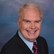# Math Solutions: Alkalinity CalculationsBy Daniel Theobald, "Wastewater Dan"

We know the importance of pH to biological wastewater treatment (covered here), but alkalinity should be considered equally important for its direct correlation to pH. Whereas pH is a measure of hydrogen ion concentration, alkalinity is the ability to neutralize acid or to absorb those hydrogen ions. Adding an alkali such as lime (calcium hydroxide) will therefore raise the pH from the acidic range (0 to 6) to the preferred, slightly alkaline range of 7 to 8 on the pH scale. Too much alkalinity — typically quantified in milligrams (mg) of calcium carbonate (CaCO3) equivalents per liter (l) of wastewater — and your pH will veer above your desired range.

This tool for operators, represented through video tutorials, offers mathematical templates to master the balancing act required for alkalinity and pH. The tool also provides definitions, explanations, and alternative applications for the calculations beyond the specific examples presented. In addition to operators, it may also serve educators, engineers, regulators, and others.

A representative alkalinity math word problem followed by exact step-by-step solutions is presented  below.

Determine the number of bags of lime needed to adjust alkalinity in the biological wastewater treatment process system. Assume 25.0 mg of nitrogen (N) oxidized in the process system, flow of 2.22 MGD, 125 mg/L alkalinity in the process system, 75 mg/L calcium carbonate (CaCO3), and 50-lb bags of lime.

Step-by-step solution

Required alkalinity (mg/L) as calcium carbonate:

Needed alkalinity (mg/L) as calcium carbonate:

Pounds of alkalinity (as calcium carbonate) needed:

Pounds of lime needed to adjust alkalinity:

Bags of lime needed to adjust alkalinity:

Playlist of 40-plus process control (including activated sludge) calculations

Playlist of 45-plus pounds-wastewater calculations

Playlist of all 150-plus Math Solutions

This is the Alkalinity Calculations presentation in my series of “Math Solutions.” If you have specific wastewater math queries, please submit a question